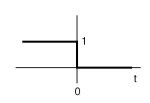# Basic operations on continuous time signal

## Basic operations on continuous time signal

In this section we will study some basic operations on continuous time signal:

### A)     Operation performed on dependent variables:

These operations include sum, product, difference, even, odd, etc.

#### 1)      Amplitude scaling:

Amplitude scaling means changing an amplitude of given continuous time signal. We will denote continuous time signal by x(t). If it is multiplied by some constant ‘B’ then resulting signal is,

y(t)= B x(t)

Example: Sketch y(t) = 5u(t)

Solution: we know that u(t) is unit step function. So if we multiply it with 5, its amplitude will become 5 and it shown as follows:

#### 2)      Sum and difference of two signals:

Consider two signals x1(t) and x2(t). Then addition of these signals is denoted by y(t)=x1(t)+x2(t). similarly subtraction is given by y(t)=x1(t)-x2(t).

Example: Sketch y(t) = u(t) – u(t – 2)

Solution: First, plot each of the portions of this signal separately

• x1(t) = u(t) …….Simply a step signal

• x2(t) = –u(t-2) ……. Delayed step signal by 2 units and multiplied by -1.

Then, move from one side to the other, and add their instantaneous values:

#### 3)      Product of two signals:

If x1(t) and x2(t) are two continuous signals then the product of x1(t) and x2(t) is,

Y(t) = x1(t)x2(t).

Example:  Sketch y(t) = u(t)·u(t – 2)

Solution: First, plot each of the portions of this signal separately

• x1(t) = u(t) _ Simply a step signal

• x2(t) = u(t-2) _ Delayed step signal

Then, move from one side to the other, and multiply instantaneous values:

#### 4)      Even and odd parts:

Even part of signal x(t) is given by,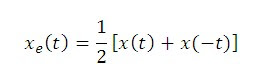And odd part of x(t) is given by,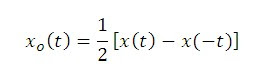### B)     Operations performed on independent variables:

#### 1)      Time shifting:

A signal x(t) is said to be ‘shifted in time’ if we replace t by (t-T). thus x(t-T) represents the time shifted version of x(t) and the amount of time shift is ‘T’ sec. if T is positive then the shift is to right (delay) and if T is negative then the shift is to the left (advance).

Example:  Sketch y(t) = u(t – 2)

solution: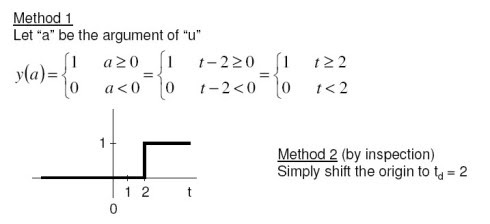#### 2)      Time scaling:

The compression or expression of a signal in time is known as the time scaling. If x(t is the original signal then x(at) represents its time scaled version. Where a is constant.

If a> 1 then x(at) will be a compressed version of x(t) and if a< 1 then it will be a expanded version of x(t).

Example:  Let x(t) = u(t) – u(t – 2). Sketch y(t) = x(t/2)

solution: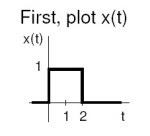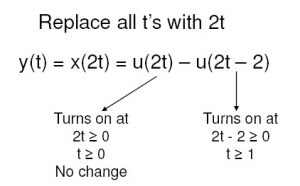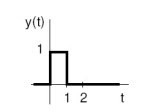3)      Time reversal (Time inversion):

Flips the signal about the y axis. y(t) = x(-t) .

Example: Let x(t) = u(t), and perform time reversal.

solution: